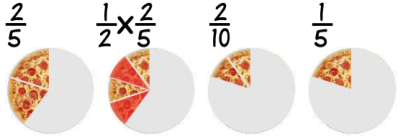# 分数乘法

### 例子： 1 2 × 2 5

、乘上面的数：

1 2  ×  2 5   =   1 × 2     =   2

、乘下面的数

1 2  ×  2 5   =   1 × 2 2 × 5   =   2 10

2 10 = 1 5

### 用披萨来做。。。。。。留意到十分之二写成五分之一会简单点吗？

### 再一个例子 1 3 × 9 16

、乘上面的数：

1 3  ×  9 16   =   1 × 9     =   9

、乘下面的数：

1 3  ×  9 16   =   1 × 9 3 × 16   =   9 48

、约简分数：

9 48 = 3 16

（这次我们把上面和下面都除以 3 来约简）

"乘分数

## 分数和整数

### 例子： 2 3  ×  5

2 3  ×  5 1

2 3  ×  5 1   =   2 × 5 3 × 1   =   10 3

### 例子： 3 ×  2 9

3    ×  2 9   =   3 × 2 9   =   6 9

6 9 = 2 3